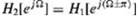# a. If h 1 [n] and h 2 [n], the impulse responses of two LTID systems are related by h 2 [n] = (-1)…

a. If h1[n] and h2[n], the impulse responses of two LTID systems are related by h2[n] = (−1)nh1,[n], then show thatHow are the frequency response spectrum H2[e] related to the H1[e].

b. If H1 [z] represents an ideal low pass filter with cutoff frequency Ωc, sketch H2[e]. What type of filter is H2[e]?